# Digital Sequential Circuits

Advertisements

We discussed various combinational circuits in earlier chapters. All these circuits have a set of output(s), which depends only on the combination of present inputs. The following figure shows the block diagram of sequential circuit.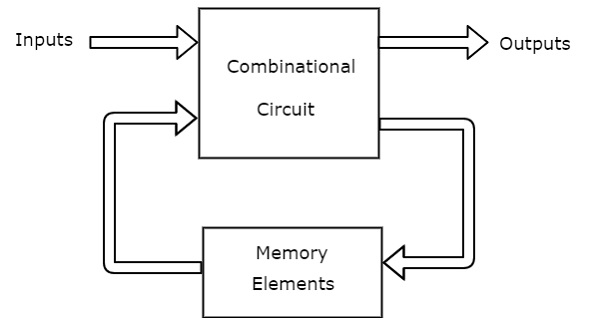This sequential circuit contains a set of inputs and output(s). The output(s) of sequential circuit depends not only on the combination of present inputs but also on the previous output(s). Previous output is nothing but the present state. Therefore, sequential circuits contain combinational circuits along with memory (storage) elements. Some sequential circuits may not contain combinational circuits, but only memory elements.

Following table shows the differences between combinational circuits and sequential circuits.

Combinational Circuits Sequential Circuits
Outputs depend only on present inputs. Outputs depend on both present inputs and present state.
Feedback path is not present. Feedback path is present.
Memory elements are not required. Memory elements are required.
Clock signal is not required. Clock signal is required.
Easy to design. Difficult to design.

## Types of Sequential Circuits

Following are the two types of sequential circuits −

• Asynchronous sequential circuits
• Synchronous sequential circuits

### Asynchronous sequential circuits

If some or all the outputs of a sequential circuit do not change (affect) with respect to active transition of clock signal, then that sequential circuit is called as Asynchronous sequential circuit. That means, all the outputs of asynchronous sequential circuits do not change (affect) at the same time. Therefore, most of the outputs of asynchronous sequential circuits are not in synchronous with either only positive edges or only negative edges of clock signal.

### Synchronous sequential circuits

If all the outputs of a sequential circuit change (affect) with respect to active transition of clock signal, then that sequential circuit is called as Synchronous sequential circuit. That means, all the outputs of synchronous sequential circuits change (affect) at the same time. Therefore, the outputs of synchronous sequential circuits are in synchronous with either only positive edges or only negative edges of clock signal.

## Clock Signal and Triggering

In this section, let us discuss about the clock signal and types of triggering one by one.

### Clock signal

Clock signal is a periodic signal and its ON time and OFF time need not be the same. We can represent the clock signal as a square wave, when both its ON time and OFF time are same. This clock signal is shown in the following figure.n the above figure, square wave is considered as clock signal. This signal stays at logic High (5V) for some time and stays at logic Low (0V) for equal amount of time. This pattern repeats with some time period. In this case, the time period will be equal to either twice of ON time or twice of OFF time.

We can represent the clock signal as train of pulses, when ON time and OFF time are not same. This clock signal is shown in the following figure.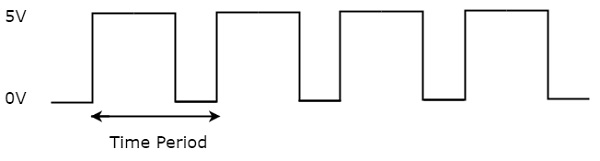In the above figure, train of pulses is considered as clock signal. This signal stays at logic High (5V) for some time and stays at logic Low (0V) for some other time. This pattern repeats with some time period. In this case, the time period will be equal to sum of ON time and OFF time.

The reciprocal of the time period of clock signal is known as the frequency of the clock signal. All sequential circuits are operated with clock signal. So, the frequency at which the sequential circuits can be operated accordingly the clock signal frequency has to be chosen.

## Types of Triggering

Following are the two possible types of triggering that are used in sequential circuits.

• Level triggering
• Edge triggering

### Level triggering

There are two levels, namely logic High and logic Low in clock signal. Following are the two types of level triggering.

• Positive level triggering
• Negative level triggering

If the sequential circuit is operated with the clock signal when it is in Logic High, then that type of triggering is known as Positive level triggering. It is highlighted in below figure.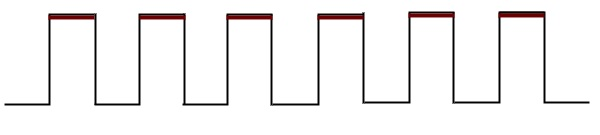If the sequential circuit is operated with the clock signal when it is in Logic Low, then that type of triggering is known as Negative level triggering. It is highlighted in the following figure.### Edge triggering

There are two types of transitions that occur in clock signal. That means, the clock signal transitions either from Logic Low to Logic High or Logic High to Logic Low.

Following are the two types of edge triggering based on the transitions of clock signal.

• Positive edge triggering
• Negative edge triggering

If the sequential circuit is operated with the clock signal that is transitioning from Logic Low to Logic High, then that type of triggering is known as Positive edge triggering. It is also called as rising edge triggering. It is shown in the following figure.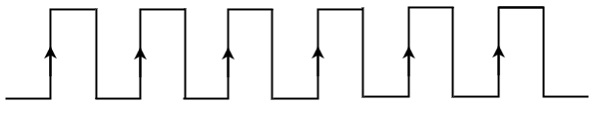If the sequential circuit is operated with the clock signal that is transitioning from Logic High to Logic Low, then that type of triggering is known as Negative edge triggering. It is also called as falling edge triggering. It is shown in the following figure.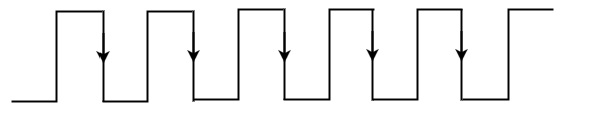In coming chapters, we will discuss about various sequential circuits based on the type of triggering that can be used in it.

Advertisements